Flip Flops can be confusing at times. Let us make it simpler.

What are flip flops?

They are bistable elements used as memory elements in sequential circuits,capable of storing 1- bit binary information

Constructing flip flops

1. Try to use the same logic gate for all type of flip flops.
2. If using NOR gate then ‘1’ means set and ‘0’ means reset.
3. If using NAND gate then ‘0’ means set and ‘1’ means reset.

There are 4 types of flip flops.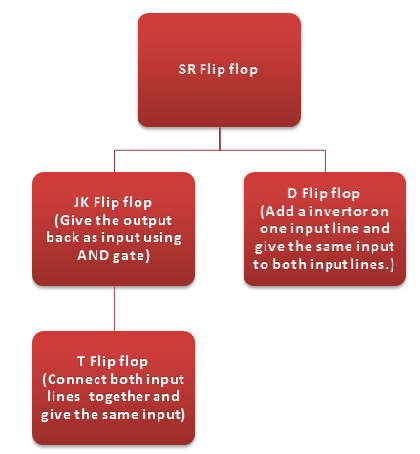This diagram shows that the basic flip flop is the Set Reset flip flop (Based on NOR gate) and every other flip flop is derived from that.The design changes are shown in the diagram.
Reasons for design change

• SR flip flop has a problem of indeterminate state which was overcome by JK flip flop.
• JK flip flop is formed by feeding the output back to the input. There arises a Race around condition which can be avoided if the given clock pulse is edge triggered or pulse triggered.
• The modified edge triggered version of SR and JK are D and T flip flops respectively.

Characteristic Equation

 Flip Flops Equation SR Qn+1=S+Qn JK Qn+1=Qn+Qn D Qn+1=D T Qn+1=Tn+Qn

Logic Diagram and Truth table

SR Flip Flop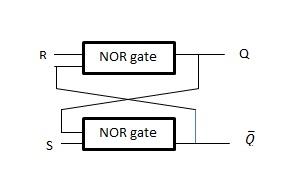S R Q Q’ 1 0 1 0 0 1 0 1 0 0 0 1 1 1 indeterminate

The flip flop is set when S=1 and when R=1 it is reset.
When both S=R=0 then the output retains the previous value. In this case it retains the value ‘0’.
S=R=1 is to be avoided.When both inputs are set to ‘1’ they make the NOR gates go to a low state.(Q=0,Q’=0). As it violates the normal logic, this condition is named as indeterminate condition.

JK Flip Flop

 J K Qn Qn+1 0 0 0 0 0 1 0 0 1 0 0 1 1 1 0 1 0 0 1 1 0 1 1 0 1 0 1 1 1 1 1 0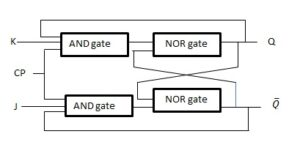The indeterminate condition which occurs in SR flip flop is overcome in this JK flip flop by feeding the output to the input again.When J=K=0, Qn+1 follows the previous state. When J=K=1, it follows Q’n.

D Flip Flop

 D Qn Qn+1 0 0 0 1 0 1 0 1 0 1 1 1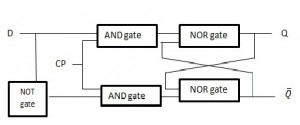Here  the flip flop is set on the positive going edges of the clock pulse(CP in the diagram).

T Flip Flop

 T Qn Qn+1 0 0 0 1 0 1 0 1 1 1 1 0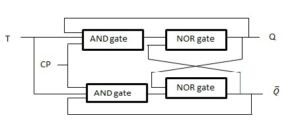There would be no change in the output when T=0 as the AND gates are disabled. When T=1 the output toggles.

Let us look into application of flip flop as counters in the next post.

Share.